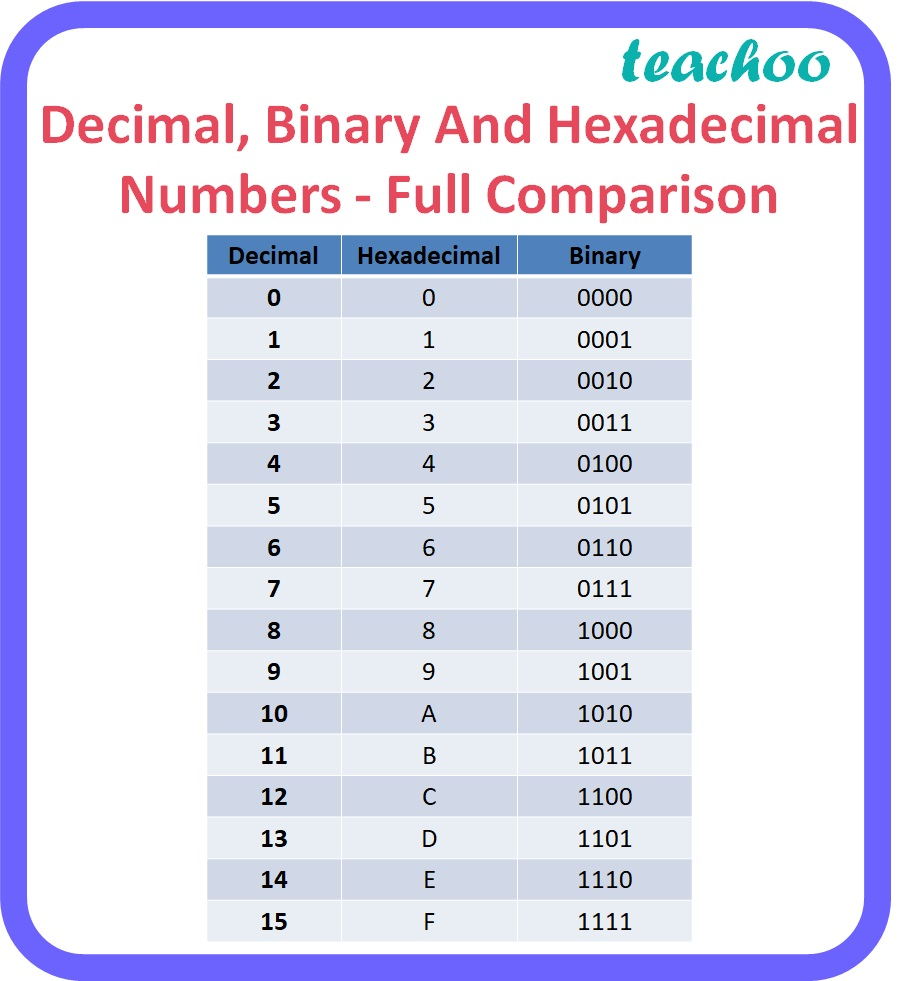3 Mark Questions

Computer Science - Class 11
Chapter 2 Class 11 - Encoding Schemes and Number System

## (b) Binary

1C6A 16

1. Decimal

1C6A 16 = 1 x 16 3 + 12 x 16 2 + 6 x 16 1 + 10 x 16 0

= 4096 + 3072 + 96 + 10

= 7274 10

Explanation:

• To obtain the decimal equivalent of a hexadecimal number, individual digits of hexadecimal number should be multiplied by powers of 16 starting with rightmost digit multiplied by 16 0 , second last digit multiplied by 16 1 , third last digit multiplied by 16 2 and so on up to the leftmost digit.
• For digits after the decimal point, the leftmost digit should be multiplied by 16 -1 and the next digit by 16 -2 and so on up to the rightmost digit.
• For alphabets A to F, the corresponding codes should be multiplied with the respective power of 16.

Here,

• A = 10
• B = 11
• C = 12
• D = 13
• E = 14
• F = 15

b. Binary

1C6A 16

 1 C 6 A 0001 1100 0110 1010

Therefore, 1C6A 10 = 0001110001101010 2

Explanation:

• To obtain the binary equivalent of a hexadecimal number, individual digits of the hexadecimal number should be converted to binary in groups of four digits as given in the table below.Learn in your speed, with individual attention - Teachoo Maths 1-on-1 Class